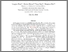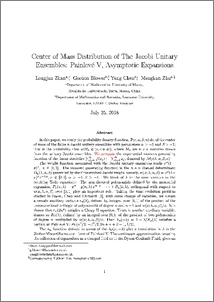# Center of mass distribution of the Jacobi unitary ensembles:Painleve V, asymptotic expansions

Zhan, Longjun and Blower, Gordon and Chen, Yang and Zhu, Mengkun (2018) Center of mass distribution of the Jacobi unitary ensembles:Painleve V, asymptotic expansions. Journal of Mathematical Physics, 59 (10). ISSN 0022-2488Preview
PDF (PVpaper)
PVpaper.pdf - Accepted Version

## Abstract

In this paper, we study the probability density function, $\mathbb{P}(c,\alpha,\beta, n)\,dc$, of the center of mass of the finite $n$ Jacobi unitary ensembles with parameters $\alpha\,>-1$ and $\beta >-1$; that is the probability that ${\rm tr}M_n\in(c, c+dc),$ where $M_n$ are $n\times n$ matrices drawn from the unitary Jacobi ensembles. We first compute the exponential moment generating function of the linear statistics $\sum_{j=1}^{n}\,f(x_j):=\sum_{j=1}^{n}x_j,$ denoted by $\mathcal{M}_f(\lambda,\alpha,\beta,n)$. The weight function associated with the Jacobi unitary ensembles reads $x^{\alpha}(1-x)^{\beta},\; x\in [0,1]$. The moment generating function is the $n\times n$ Hankel determinant $D_n(\lambda,\alpha,\beta)$ generated by the time-evolved Jacobi weight, namely, $w(x;\lambda ,\alpha,\beta )=x^{\alpha}(1-x)^{\beta}\,{\rm e}^{-\lambda\:x},\,x\in[0,1],\,\alpha>-1,\,\beta>-1$. We think of $\lambda$ as the time variable in the resulting Toda equations. The non-classical polynomials defined by the monomial expansion, $P_n(x,\lambda)= x^n+ p(n,\lambda)\:x^{n-1}+\dots+P_n(0,\lambda)$, orthogonal with respect to $w(x,\lambda,\alpha,\beta )$ over $[0,1]$ play an important role. Taking the time evolution problem studied in Basor, Chen and Ehrhardt (\cite{BasorChenEhrhardt2010}), with some change of variables, we obtain a certain auxiliary variable $r_n(\lambda),$ defined by integral over $[0,1]$ of the product of the unconventional orthogonal polynomials of degree $n$ and $n-1$ and $w(x,\lambda,\alpha,\beta )/x$. It is shown that $r_n(2\imath\/{\rm e}^{z})$ satisfies a Chazy $II$ equation. There is another auxiliary variable, denote as $R_n(\lambda),$ defined by an integral over $[0,1]$ of the product of two polynomials of degree $n$ multiplied by $w(x,\lambda)/x.$ Then $Y_n(-\lambda)=1-\lambda/R_n(\lambda)$ satisfies a particular Painlev\'{e} \uppercase\expandafter{\romannumeral 5}: $P_{\rm V}(\alpha^2/2$, $-\beta^2/2, 2n+\alpha+\beta+1,1/2)$.\\ The $\sigma_n$ function defined in terms of the $\lambda\:p(n,-\lambda)$ plus a translation in $\lambda$ is the Jimbo--Miwa--Okamoto $\sigma$-form of Painlev\'{e} \uppercase\expandafter{\romannumeral 5}. In the continuum approximation, treating the collection of eigenvalues as a charged fluid as in the Dyson Coulomb Fluid, gives an approximation for the moment generation function $\mathcal{M}_f(\lambda,\alpha,\beta,n)$ when $n$ is sufficiently large. Furthermore, we deduce a new expression of $\mathcal{M}_f(\lambda,\alpha,\beta,n)$ when $n$ is finite in terms the $\sigma$ function of this the Painlev\'{e} \uppercase\expandafter{\romannumeral 5} An estimate shows that the moment generating function is a function of exponential type and of order $n$. From the Paley-Wiener theorem, one deduces that $\mathbb{P}(c,\alpha,\beta,n)$ has compact support $[0,n]$. This result is easily extended to the $\beta$ ensembles, as long as $w$ the weight is positive and continuous over $[0,1].$

Item Type:
Journal Article
Journal or Publication Title:
Journal of Mathematical Physics
Copyright 2018 American Institute of Physics. The following article appeared in Journal of Mathematical Physics, 59 (10), 2018 and may be found at http://dx.doi.org/10.1063/1.5023128 This article may be downloaded for personal use only. Any other use requires prior permission of the author and the American Institute of Physics.
Uncontrolled Keywords:
/dk/atira/pure/subjectarea/asjc/3100/3109
Subjects:
Departments:
ID Code:
127020
Deposited By:
Deposited On:
21 Aug 2018 14:26
Refereed?:
Yes
Published?:
Published